# LOG#037. Relativity: Examples(I)

Problem 1. In the S-frame, 2 events are happening simultaneously at 3 lyrs of distance. In the S’-frame those events happen at 3.5 lyrs. Answer to the following questions: i) What is the relative speed between frames? ii) What is the temporal distance of events in the S’-frame?

Solution. i)And by simultaneity,Then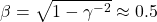ii)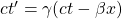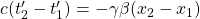since we have simultaneity implies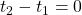. Then,Problem 2. In S-frame 2 events occur at the same point separated by a temporal distance of 3yrs. In the S’-frame,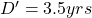is their spatial separation. Answer the next questions: i) What is the relative velocity between the two frames? ii) What is the spatial separation of events in the S’-frame?

Solution. i)with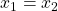As the events occur in the same point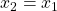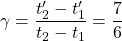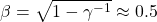ii)impliesTherefore, the second event happens 1.8 lyrs to the “left” of the first event. It’s logical: the S’-frame is moving with relative speed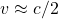for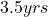.

Problem 3. Two events in the S-frame have the following coordinates in spacetime: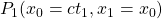, i.e.,and, i.e.,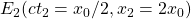. The S’-frame moves with velocity v respect to the S-frame. a) What is the magnitude of v if we want that the events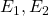were simultaneous? b) At what tmes t’ do these events occur in the S’-frame?

Solution. a)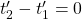and then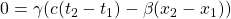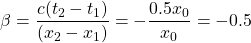b)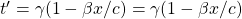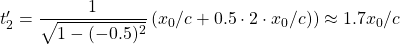Problem 4. A spaceship is leaving Earth with. When it is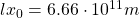away from our planet, Earth transmits a radio signal towards the spaceship. a) How long does the electromagnetic wave travel in the Earth-frame? b) How long does the electromagnetic wave travel in the space-ship frame?

For the spaceship,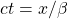and for the signal. From these equations, we get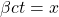and, and it yieldsand thus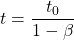for the intersection point. But,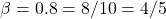and. Putting this value in the intersection point, we deduce that the intersection point happens at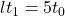. Moreover,b) We have to perform a Lorentz transformation from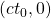to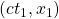, with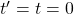.and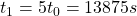. Then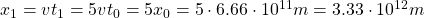. And thus, we obtain that. The Lorentz transformation for the two events readRemarks: a) Note that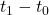and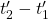differ by 3 instead of. This is due to the fact we haven’t got a time interval elapsing at a certain location but we face with a time interval between two different and spatially separated events.

b)The use of the complete Lorentz transformation (boost) mixing space and time is inevitable.

Problem 5. Two charged particles A and B, with the same charge q, move parallel with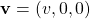. They are separated by a distance d. What is the electric force between them?In the S-frame, we obtain the Lorentz force:The same result can be obtained using the power-force (or forpower) tetravector performing an inverse Lorentz transformation.

Problem 6. Calculate the electric and magnetic field for a point particle passing some concrete point.

The electric field for the static charge is:withwhen the temporal origin coincides, i.e., at the time.  Suppose now two points that for the rest observer provide: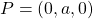and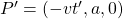. For the electric field we get: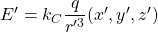and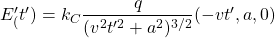Then,implies that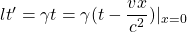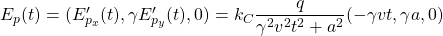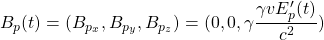There are two special cases from the physical viewpoint in the observed electric fields:

a) When P is directly above the charge q. Thenb) When P is directly in front of ( or behind) q. Then, for a=0,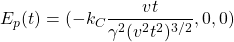Note that we have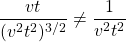if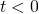.

This site uses Akismet to reduce spam. Learn how your comment data is processed.